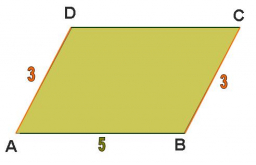# Parallelogram 65334

In a parallelogram, the sum of the lengths of the sides a+b = 234. The angle subtended by the sides a and b is 60°. The diagonal size against the given angle of 60° is u=162. Calculate the sides of the parallelogram, its perimeter, and its area.

a =  181.6916
b =  52.3084
o =  468
S =  8230.7054

### Step-by-step explanation:

Our quadratic equation calculator calculates it.

Try calculation via our triangle calculator.Did you find an error or inaccuracy? Feel free to write us. Thank you!

Tips for related online calculators
Are you looking for help with calculating roots of a quadratic equation?
Do you have a linear equation or system of equations and looking for its solution? Or do you have a quadratic equation?
Do you want to convert time units like minutes to seconds?
Cosine rule uses trigonometric SAS triangle calculator.